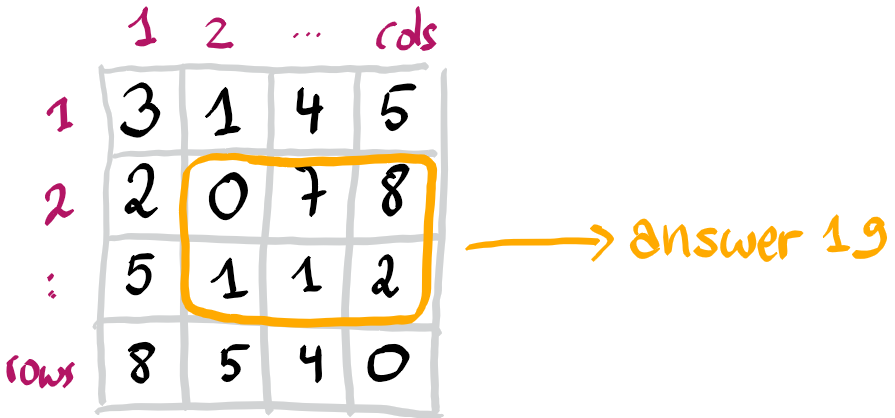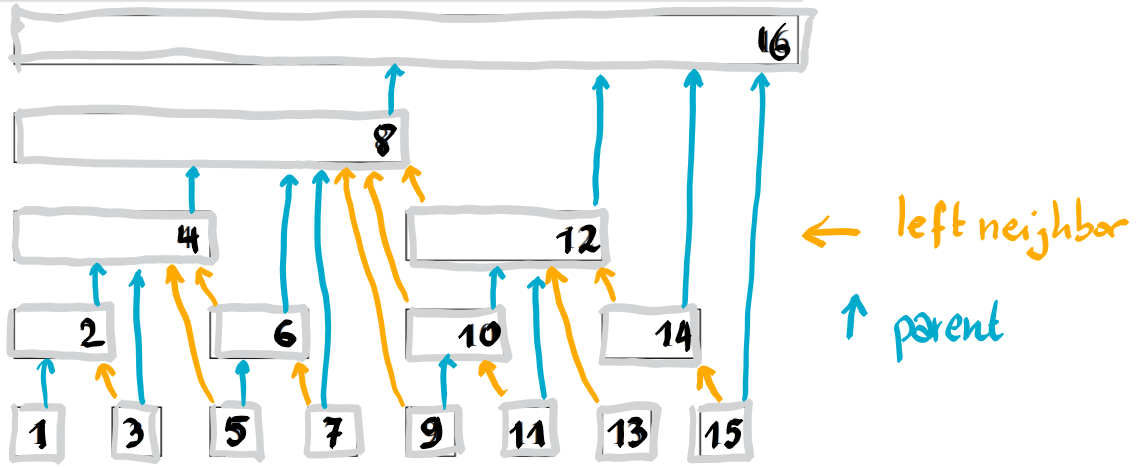# Résolution de problèmes algorithmiques

Maintain a matrix in a datastructure, allowing to update entries and to query the sum of a rectangular submatrix in time $O(\log(n)\log(m))$, where $n,m$ are the dimensions of the matrix.

# Notation

For convenience, we assume that the matrix rows and columns are numbered starting from 1.The one-dimensional variant of this problem, can be solved with a segment tree or a Fenwick tree. We solve this problem, using a two-dimensional Fenwick tree, which is also described here for instance.

# One dimensional Fenwick tree

The standard Fenwick tree maintains an array, with indices starting at 1, such that one can update entries as well query the sum of a given prefix of the array. All these operations take logarithmic time in the size of the array.

The idea is to store the array in a tree. Every node is responsible for some interval of indices and contains the sum of the corresponding entries. Every node j is responsible of for an interval of the form [i,j] for some i. Its left neighbor is the node i-1 if i>1, otherwise the node j has no left neighbor. In addition, every node has a parent in the tree, except the root of course.Parents and left neighbors are easy to determine. Let k be the smallest power of two which is present in the binary decomposition of the number j. Then the left neighbor (if any) of node j is j-k, and its parent (if j is not the root) is j+k. The integer k can be easily determined from j by the expression j & -j. You can easily convince yourself, by writing j and -j in binary.

Now in order to determine the prefix sum of the array up to index j, one needs to return the sums stored in the nodes starting from node j, and following all left neighbors. The intervals for which the nodes are responsible for, partition the interval [1,j].

In order to add a value to a specific entry j, one needs first to add this value to the node j and then iteratively to all parent nodes.

Our implementation of this structure can be found here.

# Two dimensional Fenwick tree

The standard Fenwick tree is only conceptually a tree and in fact is stored as an array. Similar the two-dimensional Fenwick tree is stored as a matrix. The idea is that there is a first tree on the rows, and each of its entries is another Fenwick tree on the columns. The update and query operators are therefore similar to the one dimensional case, excepted that now there are nested loops, the outer one for the rows, and the inner one for the colums.

A query asks for the sum of the upper left rectangular submatrix ending at given coordinates (i,j). Using the inclusion-exclusion principle, one can determine the sum of any rectangular submatrix.